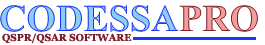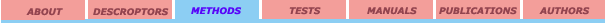Multilinear regression Selection of descriptors Multivariate methods

## Multivariate methods

The principal component analysis (PCA) is generally described as an ordination technique for describing the variation in a multivariate data set.[1, 2, 3] The first axis (the first principal component, or PC1) describes the maximum variation in the whole data set; the second describes the maximum variance remaining, and so forth, with each axis orthogonal to the preceding axis. Principal components are eigenvectors of a covariance X’X or correlation X’Y matrix. The number of principal components that can be extracted will typically exceed the maximum of the number of Yand Xvariables.

The principal component analysis and factor analysis are based on the separation of the original matrix X into two matrixes: factor scores matrix T and loading matrix Q. In matrix form: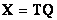The columns in a factor score matrix are linear independent. Usually, the columns in X and Y matrix are centered (by subtracting their means) and scaled (by dividing by their standard deviations). Suppose we have a data set with response variables Y (in matrix form) and a large number of predictor variables X (in matrix form), some of which are highly correlated. A regression, using factor extraction for this type of data, computes the factor score matrix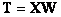for an appropriate weight matrix W, and then considers the linear regression modelwhere Q is a matrix of regression coefficients (loadings) for T, and E is an error (noise) term. Once the loadings Q are computed, the above regression model is equivalent to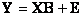which can be used as a predictive regression model.

The factor scores and loadings can be obtained in many different ways. NIPALS algorithm was developed in 1923,  later modified in 1966,  and SIMPLS algorithm   resulted from work by de Jong in 1993. Singular value decomposition is another commonly used method for calculating scores and loading.

Principal components regression (PCR) and partial least squares (PLS) regression differ in the methods used for extracting factor scores. PLR produces the weight matrix W reflecting the covariance structure between the predictor variables, while PLS regression produces the weight matrix W reflecting the covariance structure between the predictor and response variables. In PLSregression, prediction functions are represented by factors extracted from the Y'XX'Y matrix.

For establishing the model, PLS regression produces a weight matrix W for X such that T=XW, i.e., the columns of W are weight vectors for the X columns producing the corresponding factor score matrix T. These weights are computed so that each of them maximizes the covariance between responses and the corresponding factor scores. Ordinary least squares procedures for the regression of Y on T are then performed to produce Q, the loadings for Y(or weights for Y) such that Y=TQ+E. Once Q is computed, we have Y=XB+E, where B=WQ, and the prediction model is complete.

One additional matrix which is necessary for a complete description of partial least squares regression procedures is the factor loading matrix P which gives a factor model X=TP+F, where F is the unexplained part of the X scores.

References:

1. Manly, B. F. J. Multivariate Statistical Methods. A Primer. London-NY: Chapman and Hall, 1986.

2. Nilsson, J. Multiway calibration in 3D QSAR: applications to dopamine receptor ligands; Groningen: University Library Groningen, 1998, Online Resource.

3. Fisher, R.; MacKensie, W. Journal of Agricaltural Science 1923, 13, 311-320.
4. Wold, H., In: Research papers in Statistics, ed. David, F., NY: Wiley & Sons, 1966, pp.411-444.
5. de Jong, S. SIMPLS: An Alternative Approach to Partial Least Squares Regression, Chemometrics and Intelligent Laboratory Systems 1993, 18, 251-263
6. Mandel, J. American Statistician 1982, 36, 15-24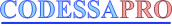University of Florida 2001. All rights reserved